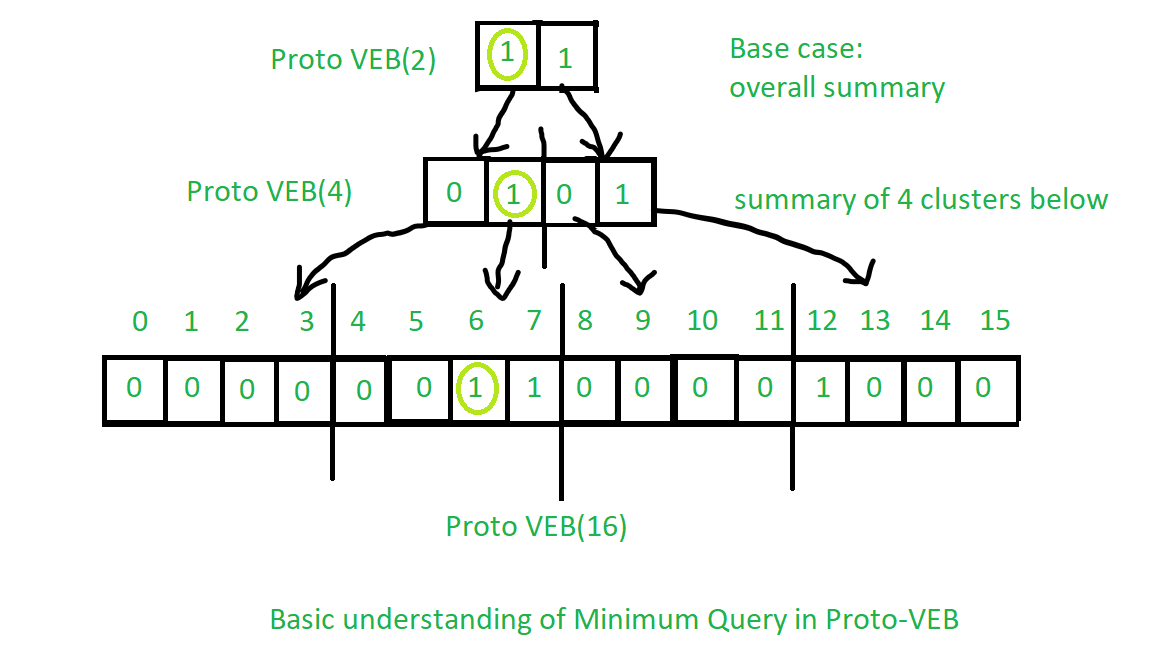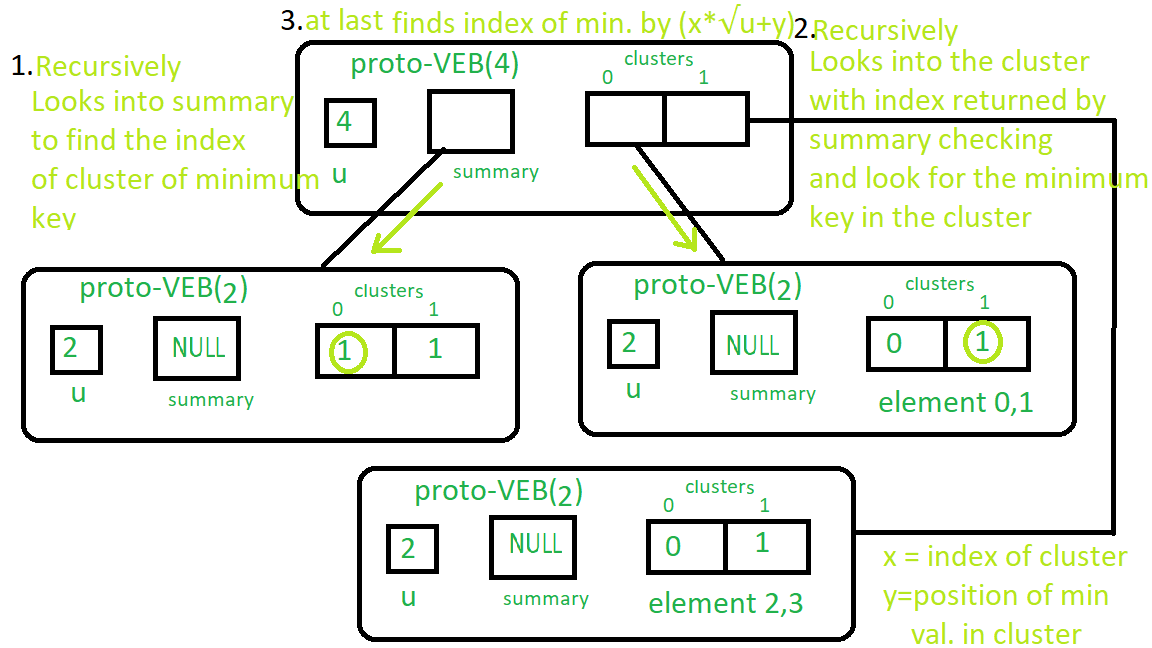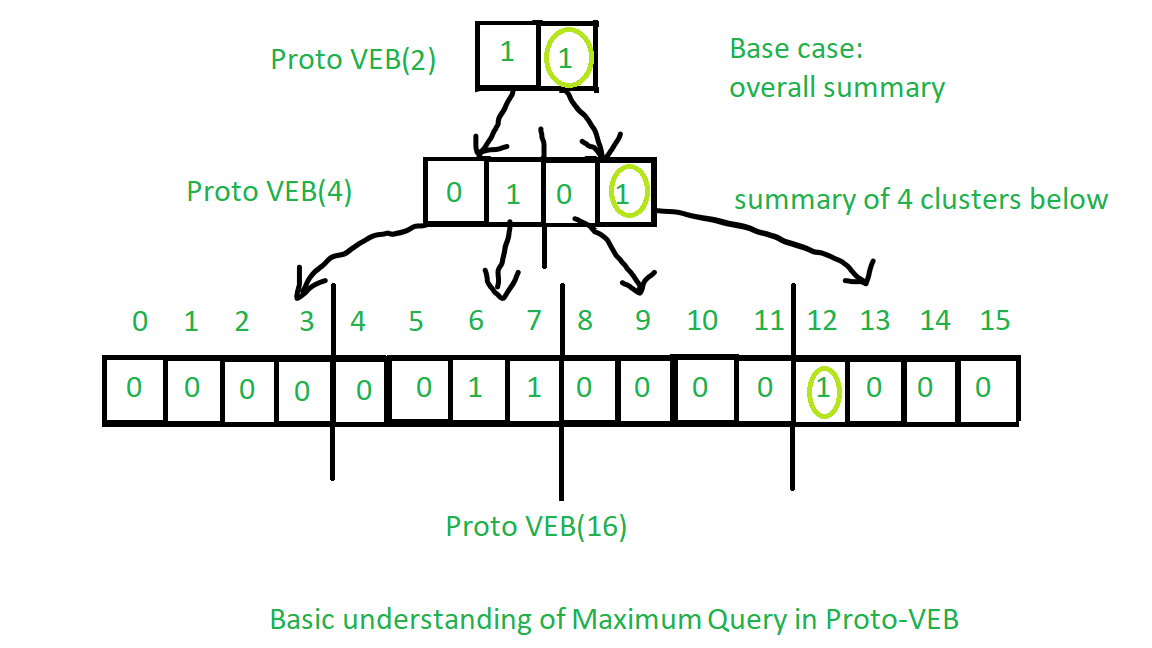Related Articles

# Proto Van Emde Boas Tree | Set 5 | Queries: Minimum, Maximum

• Last Updated : 04 Oct, 2021

Please check previous sets of Proto Van Emde Boas Tree article first. It is highly recommended.

Procedure for finding minimum:

1. Base Case: If the size of Proto-VEB is 2 then we will return smallest key present in the cluster if no keys present then we will return -1 as the symbol that no keys are present.
2. Recursion:
• We will start recursion over summary until we reach the first true value(In the code, first not nullptr in the summary cluster) which shows that there is a key present in that cluster.
• Now We will find the position of the key in that cluster using again recursively call over a cluster to find the first true value (In the code, first not nullptr in the cluster) in a cluster as we have done above.

• Finally, we will return the index of that key according to cluster number we get from procedure over summary and position we get from the procedure over the cluster in the last step.

See the image below for the basic understanding of the operation:
Observe the light green circles from top to bottom:See the image below for real Proto – VEB minimum operation:
Follow the instructions in order of numberings.You can easily get the idea of Maximum from the minimum procedure. See the image below:
Observe the light green circles from top to bottom:Implementation of the above algorithm:

## C++

 // C++ implementation of the approach#include using namespace std; class Proto_Van_Emde_Boas {public:    // Total number of keys    int universe_size;     // Summary    Proto_Van_Emde_Boas* summary;     // Clusters array of Proto-VEB pointers    vector clusters;     int root(int u)    {        return (int)sqrt(u);    }     // Function to return cluster numbers    // in which key is present    int high(int x)    {        return x / root(universe_size);    }     // Function to return position of x in cluster    int low(int x)    {        return x % root(universe_size);    }     // Function to return the index from    // cluster number and position    int generate_index(int cluster, int position)    {        return cluster * root(universe_size) + position;    }     // Constructor    Proto_Van_Emde_Boas(int size)    {        universe_size = size;         // Base case        if (size <= 2) {             // Set summary to nullptr as there is no            // more summary for size 2            summary = nullptr;             // Vector of two pointers            // nullptr in starting            clusters = vector(size, nullptr);        }        else {             // Assigning Proto-VEB(sqrt(u)) to summary            summary = new Proto_Van_Emde_Boas(root(size));             // Creating array of Proto-VEB Tree pointers of size sqrt(u)            // first all nullptrs are going to assign            clusters = vector(root(size), nullptr);             // Assigning Proto-VEB(sqrt(u)) to all its clusters            for (int i = 0; i < root(size); i++) {                clusters[i] = new Proto_Van_Emde_Boas(root(size));            }        }    }}; // Function that returns true if the// key is present in the treebool isMember(Proto_Van_Emde_Boas* helper, int key){     // If key is greater then universe_size then    // returns false    if (key >= helper->universe_size)        return false;     // If we reach at base case    // the just return whether    // pointer is nullptr then false    // else return true    if (helper->universe_size == 2) {        return helper->clusters[key];    }    else {         // Recursively go deep into the        // level of Proto-VEB tree using its        // cluster index and its position        return isMember(helper->clusters[helper->high(key)],                        helper->low(key));    }} // Function to insert a key in the treevoid insert(Proto_Van_Emde_Boas*& helper, int key){    // If we reach at base case    // then assign Proto-VEB(1) in place    // of nullptr    if (helper->universe_size == 2) {        helper->clusters[key] = new Proto_Van_Emde_Boas(1);    }    else {         // Recursively using index of cluster and its        // position in cluster        insert(helper->clusters[helper->high(key)],               helper->low(key));         // Also do the same recusion in summary VEB        insert(helper->summary, helper->high(key));    }} // Function to return the minimum key from the treeint minimum(Proto_Van_Emde_Boas* helper){    // Base case choses the least key    // present in the cluster    if (helper->universe_size == 2) {        if (helper->clusters) {            return 0;        }        else if (helper->clusters) {            return 1;        }         // No keys present then return -1        return -1;    }    else {         // Recursively find in summary for        // first 1 present in Proto-VEB        int minimum_cluster = minimum(helper->summary);        int offset;         // If no key is present in        // the cluster then return -1        if (minimum_cluster == -1) {            return -1;        }        else {             // Recursively find the position of the key            // in the minimum_cluster            offset = minimum(helper->clusters[minimum_cluster]);             // Returns overall index of minimum key            return helper->generate_index(minimum_cluster, offset);        }    }} // Function to return the maximum key from the treeint maximum(Proto_Van_Emde_Boas* helper){     // Return the maximum key present in    // the cluster    if (helper->universe_size == 2) {        if (helper->clusters) {            return 1;        }        else if (helper->clusters) {            return 0;        }         // Return -1 if no keys present in the        // cluster        return -1;    }    else {         // Recursively find the last 1 present        // in the summary        int maximum_cluster = maximum(helper->summary);        int offset;         // If no key is present in        // the cluster then return -1        if (maximum_cluster == -1) {            return -1;        }        else {             // Recursively find the position of the key            // in the maximum_cluster            offset = maximum(helper->clusters[maximum_cluster]);            return helper->generate_index(maximum_cluster, offset);        }    }} // Function to delete a key from the treevoid pveb_delete(Proto_Van_Emde_Boas*& helper, int key){     // Base case: If the key is present    // then make it nullptr    if (helper->universe_size == 2) {        if (helper->clusters[key]) {            delete helper->clusters[key];            helper->clusters[key] = nullptr;        }    }    else {         // Recursive delete to reach at the base case        pveb_delete(helper->clusters[helper->high(key)], helper->low(key));         bool isanyinCluster = false;         // Iterate over the cluster of keys to check whether        // any other key is present within that cluster        // If yes then we should not update summary to 0        // else update summary to 0        for (int i = helper->high(key) * helper->root(helper->universe_size);             i < (helper->high(key) + 1) * helper->root(helper->universe_size);             i++) {             // If member is present then break the loop            if (isMember(helper->clusters[helper->high(key)], i)) {                isanyinCluster = true;                break;            }        }         // If no member is present then        // update summary to zero        if (isanyinCluster == false) {            pveb_delete(helper->summary, helper->high(key));        }    }} // Driver codeint main(){    Proto_Van_Emde_Boas* hello = new Proto_Van_Emde_Boas(4);     cout << boolalpha;     insert(hello, 2);     insert(hello, 3);     cout << minimum(hello) << endl;     cout << maximum(hello) << endl;}

Both Minimum and Maximum query runs in O(log2(u)) time complexity.
Recurrence Relation:

T(u) = 2T() + O(1)

Attention reader! Don’t stop learning now. Get hold of all the important DSA concepts with the DSA Self Paced Course at a student-friendly price and become industry ready.  To complete your preparation from learning a language to DS Algo and many more,  please refer Complete Interview Preparation Course.

In case you wish to attend live classes with experts, please refer DSA Live Classes for Working Professionals and Competitive Programming Live for Students.

My Personal Notes arrow_drop_up